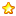# 51单片机zigbee通信C语言代码 E18_MS1+DS18B2023 |0 | 2021-5-5 23:11:02 | 显示全部楼层 |阅读模式
1、基本实现方案：
主机：单片机模拟单总线协议与DS18B20通信采集温度值，并通过串口将数据发送给zigbee模块。
从机：单片机通过串口接收zigbee接收到的温度值，然后将温度值送到模拟iic协议的数据区，并在显示器上显示出来。
2、使用到元件；
Stc89c52单片机、11.0592Mhz晶振、22pf瓷片电容、10k电阻、10uf电解电容、按键。
Zigbee 通信模块E18_MS1_PCB
DS18B20温度传感器
Oled12864显示器 0.96寸iic通信

TM16374位数码管显示模块
3、电路原理图：4、电路方案：

从机电路由单片机最小系统、zigbee无线通信模块、oled显示模块、蜂鸣器报警电路组成。

注意事项：51单片机只有一个串口（P3.0,P3.1），下载程序端口与zigbee通信端口复用，会导致zigbee连接失败，在打开电源之前将串口引脚上的跳线帽拔掉，在单片机完成初始化后再将跳线帽插回。（我估计是这个问题，但也没有好的解决办法，希望各路大师提提意见）1. #include <REG52.H>
2. #include <stdio.h>
3. #include "oled.h"
4. #include "bmp.h"

5. #define uchar unsigned char
6. #define uint unsigned int
7.
8. sbit  AT_MODE = P2^3; //zigbee通信模式选择
9. sbit  REST    = P1^7; //zigbee复位引脚
10. sbit  PBB     = P2^4; //蜂鸣器

11. uchar flag = 0;
12. uchar index = 0;
13. uchar data c ="0000";
14. uchar code str[]="123789";
15. uchar num_10,num_1,num_0;

16. void Send_string(uchar *c)
17. {
18. while(*c != '!')
19. {
20.   SBUF=*c;
21.   c++;
22.   while(TI==0);
23.   TI=0;
24. }
25. }

26. void Send_data(uchar str)
27. {
28.         SBUF=str;
29.         while(TI==0);
30.         TI=0;
31. }

32. void Send_str(uchar *s)
33. {
34.         while(*s)
35.         {
36.          Send_data(*s++);
37.     }
38. }
39. void Send_init()
40. {
41.         EA=1;ES=1;                    //开总中断和串行口中断
42.         TMOD = 0x20;            //设置定时计数器位方式2
43.         TH1  = 0xfd;            // 设置波特率为9600
44.         TL1  = 0xfd;
45.         TR1  = 1;                    //启动定时计数器
46.         SCON = 0x50;            //设置串口为方式2
47.         PCON = 0x00;            //波特率不加倍                  AT+MODE=?
48. //        TI=1;
49. }

50. void zigbee_init()
51. {
52.   AT_MODE = 1;        //AT指令模式
53.   Send_str("AT+UART=2");   //设置波特率9600
54.   Delay_1ms(1000);
55.   Send_str("AT+DEVE=E");      //终端 模式
56.   Delay_1ms(1000);
57.   Send_str("AT+MODE=3");    //协议模式
58.   Delay_1ms(1000);
59.   Send_str("AT+PANID=55AA");//设置ID号
60.   Delay_1ms(1000);
61.   Send_str("AT+RESET");           //复位
62.   Delay_1ms(5000);
63. }

64. void main(void)
65. {
66.                 u8 t,temp;
67.                  t=' ';
68.         REST = 0;
69.             Delay_1ms(100);
70.
71.                 OLED_Init();                        //初始化OLED
72.                 Send_init();
73.                 zigbee_init();
74.         REST = 1;
75.             //显示图片
76.                 OLED_DrawBMP(0,0,128,8,BMP1);  //图片显示(图片显示慎用，生成的字表较大，会占用较多空间，FLASH空间8K以下慎用)
77.                 Delay_1ms(2000);
78.                  OLED_Clear();
79.
80.                 while(1)
81.                 {

82.                   num_10= c;
83.                   num_1 = c;
84.                   num_0 = c;
85.
86.                         OLED_ShowString(0,0,"DS18B20 zigbee",16);
87.                         OLED_ShowString(0,3,"TEMP:",16);
88.                         OLED_ShowChar(50,3,num_10,16);
89.                         OLED_ShowChar(58,3,num_1,16);
90.                         OLED_ShowChar(68,3,'.',16);
91.                         OLED_ShowChar(76,3,num_0,16);

92.                         //temp = ((num_10-31)*10)+(num_1-31);
93.                         if(num_10 >= '3')PBB=1;  //温度大于30 开蜂鸣器
94.                         else PBB=0;
95.
96.                 }
97. }

98. //串口接收中断函数
99. void Serial_INT() interrupt 4
100. {
101. if(RI==0) return;
102. ES=0;        //关闭串口中断
103. RI=0;        //清接收中断标志
104. c[index++]=SBUF;
105.     if(SBUF == '!')
106.         {
107.       flag = 1;
108.           index= 0;
109.         }
110. ES=1;
111. }

 本版积分规则 回帖后跳转到最后一页302主题 124积分E币
124